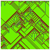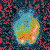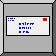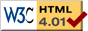# Back Propagation

(Demonstration of Back-Propagation for Project 4)

The applet requires Java 5 or higher. Java must be enabled in your browser settings. Mac users must have Mac OS X 10.4 or higher. Windows and Linux users may obtain the latest Java from Oracle's Java site.

## WHAT IS IT?

This model demonstrates back-propagation neural-network learning on several problems.

In the future this section will give a general understanding of what the model is trying to show or explain.

## HOW IT WORKS

This section will explain what rules the agents use to create the overall behavior of the model.

## HOW TO USE IT

This section will explain how to use the model, including a description of each of the items in the interface tab.

INPUTS - sets the number of inputs to the network. This is forced to the correct value by selecting an EXPERIMENT.

EXPERIMENT - selects the experiment to perform.

ENTER ARCHITECTURE - allows you to enter a list of the number of neurons in each hidden layer. For example, entering “[4 2]” puts 4 neurons in the first hidden layer and 2 in the second hidden layer.

ARCHITECTURE - displays the defined architecture, including the number of inputs (as defined by INPUTS) and the number of outputs (always 1 in this model).

SETUP - constructs a neural net with the defined architecture. The interconnection weights are randomized.

RANDOMIZE WEIGHTS - randomizes the weights. This is not necessary immediately after SETUP, but it can be used to retrain the net with different starting weights or learning parameters.

TRAINING SAMPLES - the number of sample input-output pairs used to train the neural net.

TEST SAMPLES - the number of samples used to test the net for generalization during training. It is generally a good idea to stop training when the error on the test samples begins to increase.

VALIDATION SAMPLES - the number of samples used to test the net after training has been completed.

GENERATE DATA - generates the specified numbers of training, test, and validation sample input-output pairs.

ETA - the learning rate (0.2 is a good choice)

ALPHA - determines the slope of the sigmoid function at the origin, which is ALPHA/4. ALPHA = 1 is a reasonable choice.

ONE EPOCH - trains the network for one epoch (one pass through all the training samples).

TRAIN - continuously trains, epoch after epoch, the neural net.

VALIDATE - tests the trained neural net on the validation data. It is allowed to continue training after a validation test.

ERROR ON LAST INPUT - displays the squared error on last sample processed.

TRAINING ERROR - displays the average squared error over the training data on the last epoch.

TESTING ERROR - displays the average squared error over the testing data on the last epoch.

VALIDATION ERROR - displays the average squared error over the validation data from the last VALIDATE request.

GRAPH - displays the training error (black) and testing error (red) as a function of the epoch.

PLOTTING? - For Problems 1, 3, and XOR, displays the training, test, and validation data for a brief time after GENERATE DATA is pressed, displays the network outputs for the training and test samples during training, and displays the network outputs for the validation data when VALIDATE is requested. This allows the learned behavior to be compared with the training data. The samples are displayed as scaled patch colors. Plotting is automatically turned off for Problem 2, since the function is three-dimensional.

## THINGS TO NOTICE

This section will give some ideas of things for the user to notice while running the model.

## THINGS TO TRY

This section will give some ideas of things for the user to try to do (move sliders, switches, etc.) with the model.

## EXTENDING THE MODEL

This section will give some ideas of things to add or change in the procedures tab to make the model more complicated, detailed, accurate, etc.

## NETLOGO FEATURES

This section will point out any especially interesting or unusual features of NetLogo that the model makes use of, particularly in the Procedures tab. It might also point out places where workarounds were needed because of missing features.

## RELATED MODELS

This section will give the names of models in the NetLogo Models Library or elsewhere which are of related interest.

## CREDITS AND REFERENCES

To refer to this model in academic publications, please use: MacLennan, B.J. (2008). NetLogo Back-Propagation model. http://www.cs.utk.edu/~mclennan. Dept. of Electrical Engineering & Computer Science, Univ. of Tennessee, Knoxville.

# CODE

```globals [
architecture     ; list of number of neurons in each layer
weight           ; list of weight matrices
state            ; list of state vectors
training-data    ; list of training pairs
test-data        ; list of test pairs
validation-data  ; list of validation pairs
lwbX upbX        ; bounds on X values
lwbY upbY        ; bounds on Y values
lwbZ upbZ        ; bounds on Z values (if used)
err-last-input   ; error on the last pattern processes
training-error   ; average error over training data
testing-error    ; average error over test data
validation-error ; average error over validation data
epoch            ; epoch number
]

to setup    ;;;; setup for an experiment ;;;;
set-inputs
set architecture fput inputs but-first architecture ; ensure correct number of inputs
set epoch 0
clear-outputs
reset-ticks
end

to clear-outputs
clear-all-plots
clear-patches
set err-last-input 0
set training-error 0
set testing-error 0
set validation-error 0
end

to set-inputs ; set number of inputs and bounds appropriate for the problem
ifelse Experiment = "Problem 1" [
set inputs 2
set lwbX -2 set upbX 2
set lwbY -2 set upbY 2
]
[ ifelse Experiment = "Problem 2" [
set inputs 3
set lwbX -2 set upbX 2
set lwbY -2 set upbY 2
set lwbZ -2 set upbY 2
set plotting? false ; plotting not allowed on Problem 2
]
[ ifelse Experiment = "Problem 3" [
set inputs 2
set lwbX -4 set upbX 10
set lwbY -6 set upbY 6
]
[ ifelse Experiment = "XOR" [
set inputs 2
set lwbX -2 set upbX 2
set lwbY -2 set upbY 2
]
[ ] ] ] ]
end

to enter-architecture
"Enter neurons in each hidden layer, e.g., [4 3 6]"
set-inputs
set architecture fput inputs lput 1 hidden-numbers
randomize-weights
end

to randomize-weights
set weight (map [ random-weight-matrix ?1 ?2 ] ; matrix from ?1 neurons to ?2 neurons
(but-last architecture) (but-first architecture))
clear-outputs
end

to-report random-weight-matrix [m n] ; from m neurons (+ bias) to n neurons
report n-values n [ n-values (m + 1) [ -0.1 + random-float 0.2 ] ]
end

to generate-data
set training-data n-values training_samples [ random-pair ]
set test-data n-values test_samples [ random-pair ]
set validation-data n-values validation_samples [ random-pair ]
wait 3
clear-patches
end

to-report random-pair ; generate random input output pair for training, testing, or validation
let ranX (random-input lwbX upbX)
let ranY (random-input lwbY upbY)
let pair []
ifelse Experiment = "Problem 1"
[ set pair list (list ranX ranY) Problem1 ranX ranY ]
[ ifelse Experiment = "Problem 2"
[ let ranZ (random-input lwbZ upbZ)
set pair list (list ranX ranY ranZ) Problem2 ranX ranY ranZ
]
[ ifelse Experiment = "Problem 3"
[ set pair list (list ranX ranY) Problem3 ranX ranY ]
[ ifelse Experiment = "XOR"
[ set pair list (list ranX ranY) XOR-problem ranX ranY ]
[ ] ] ] ]
if plotting? [ plot-pair (first pair) (item 1 pair) ]
report pair
end

to-report random-input [lwb upb] ; generate random number in specified bounds
report lwb + random-float (upb - lwb)
end

to-report Problem1 [x y]
report (1 + sin (90 * x) * cos (90 * y)) / 2
end

to-report Problem2 [x y z]
report (x ^ 2 / 2 + y ^ 2 / 3 + z ^ 2 / 4) * 3 / 13
end

to-report Problem3 [x y] ; two overlapping Gaussians with 1/0 outputs
let A_xy A-distribution x y
let B_xy B-distribution x y
report ifelse-value (A_xy >= random-float (A_xy + B_xy))  
end

to-report A-distribution [x y]
report exp(-0.5 * (x * x + y * y)) / (2 * pi)
end

to-report B-distribution [x y]
report exp(-0.125 * ((x - 2) ^ 2 + y * y)) / (8 * pi)
end

to-report XOR-problem [x y]
report ifelse-value (x > 0 xor y > 0)  
end

to train-one-epoch
let total-training-error 0
foreach shuffle training-data [ ; ? = an input-output pair
set total-training-error total-training-error + errorval ?
back-propagate ?
]
set training-error total-training-error / length training-data
set testing-error mean-error test-data
set epoch epoch + 1
set-current-plot-pen "training error"  plot training-error
set-current-plot-pen "testing error"   plot testing-error
end

to validate
set validation-error mean-error validation-data
end

to-report mean-error [data]
report mean map [errorval ?] data
end

let input first sample-pair
let output forward-pass input
set err-last-input difference output (last sample-pair)
if plotting? [ plot-pair input output ]
report err-last-input
end

to-report difference [output target] ;; squared error
report (output - target) ^ 2
end

to-report forward-pass [input-vector]
let prev-layer fput 1 input-vector ; prepend bias value
set state (list prev-layer)        ; start list of state vectors (one per layer)
foreach weight [                   ; ? = weight matrix between layers
let local-fields mat-vec-prod ? prev-layer
set prev-layer fput 1 map [sigmoid ?] local-fields ; prepend bias value
set state lput prev-layer state
]
report last last state ; there is only one output neuron
end

to-report sigmoid [x]
report 1 / (1 + exp(- alpha * x)) ; alpha/4 = slope at x=0
end

to back-propagate [sample]
let output last last state ; only one output neuron
let target last sample
let delta-output 2 * alpha * output * (1 - output) * (target - output)
let deltas (list delta-output) ; begin list of vectors of delta values with output layer
let Delta-output-weights outer-product deltas map [eta * ?] last butlast state
let Delta-weights (list Delta-output-weights) ; begin list weight-change matrices
; the following could be more efficient (but less clear) by reversing the state and
; weight lists once each
(foreach                            ; lists are reversed to backwards through the layers
(reverse butlast butlast state)   ; ?1 = preceding state layer
(reverse butlast weight)          ; ?2 = preceding weight layer
(reverse butfirst butlast state)  ; ?3 = hidden state layer
(reverse butfirst weight) [       ; ?4 = next weight layer
set deltas compute-deltas ?3 ?4 deltas ; compute vector of delta values for this layer
let Delta-hidden-weights outer-product deltas map [eta * ?] ?1 ; weight-change matrix
set Delta-weights fput Delta-hidden-weights Delta-weights
])
set weight
(map [ (map [ (map [?1 + ?2] ?1 ?2) ] ?1 ?2) ] weight Delta-weights) ; sequence of matrix additions
end

to-report compute-deltas [states weights deltas] ; compute deltas for one layer
;; discard delta for bias neuron when computing deltas:
report butfirst (map [ alpha * ?1 * (1 - ?1) * ?2 ] states (vec-mat-prod deltas weights))
end

to plot-pair [input output]
let x round (max-pxcor * first input / upbX)
let y floor (max-pycor * item 1 input / upbY)
set pcolor scale-color yellow output 0 1
]
end

;;; Vector and Matrix Operations ;;;

; Matrices are represented as a list of rows, each of which is a list (i.e., row-major order)

to-report inner-product [U V]
report sum (map [?1 * ?2] U V)
end

to-report mat-vec-prod [M V] ; matrix-vector product
report map [inner-product ? V] M
end

to-report vec-mat-prod [U M] ; vector-matrix product
report map [ inner-product U column ? M ]
n-values (length first M) [?]
end

to-report column [j M] ; report column-j of row-major matrix M
report map [ item j ? ] M
end

to-report outer-product [U V]
report map [scaler-product ? V] U
end

to-report scaler-product [x V] ; product of scalar and vector
report map [x * ?] V
end
```Return to CS 420/427/527 home pageReturn to MacLennan's home pageSend mail to Bruce MacLennan / MacLennan@utk.eduThis page is web.eecs.utk.edu/~mclennan/Classes/420/NetLogo5.0/Back-Propagation.html
Last updated: 2015-03-30.